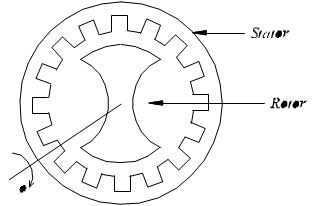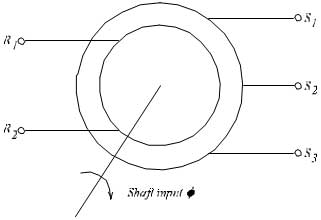# Synchro Transmitter and Receiver

Spread the love

### INTRODUCTION

The term synchro is a generic name for a family of inductive devices which works on the principle of a rotating transformer (Induction motor). The trade names for synchronous are Selsyn, Autosyn and Telesyn. Basically they are electro mechanical devices or electromagnetic transducer which produces an output voltage depending upon angular position of the rotor.

A Synchro system is formed by interconnection of the devices called the Synchro Transmitter and the synchro control transformer. They are also called as synchro pair. The synchro pair measures and compares two angular displacements and its output voltage is approximately linear with angular difference of the axis of both the shafts. They can be used in the following two ways.

i.   To control the angular position of load from a remote place / long distance.

ii.   For automatic correction of changes due to disturbance in the angular position of the load.

### SPECIFICATIONS

☞ransformer – 230/50V AC

☞Digital Voltmeter – (0-300V)AC

### Synchro Transmitter

☞Input Rotor voltage – 50VAC

☞ Output Stator Voltage – 34VAC (max)

### Synchro Receiver

☞Stator Voltage – 34V AC (max)

☞Rotor Voltage – 35VAC

### SYNCHRO TRANSMITTER

The constructional features, electrical circuit and a schematic symbol of Synchro Transmitter are shown in figure-2. The two major parts of Synchro Transmitters are stator and rotor.The stators identical to the stator of three phase alternator. It is made of laminated silicon steel and slotted on the inner periphery to accommodate a balance three phase winding. The stator winding is concentric type with the axis of the three coil 120° apart. The stator winding is star connected(Y – connection).

The rotor is of dumb bell construction with a single winding. The ends of the rotor winding are terminated on two slip rings. A single phase AC excitation voltage is applied to the rotor through the slip rings.

### Working Principles

When the rotor is excited by AC voltage, the rotor current flows, and a magnetic field is produced. The rotor magnetic field induces an emf in the stator coil by transformer action. The effective voltage induced in any stator coil depends upon the angular position of the coils axis with respect to rotor axis.Figure – Constructional Features of Synchro TransmitterFigure – 2c Electrical Circuit (Synchro Transmitter)Where,

Let    er =  Instantaneous value of AC voltage applied to rotor.

e ,e ,es1s2 s3  =  Instantaneous value of emf induced in stator coils S ,S , S with respect to12 3 neutral respectively.

Er  =  Maximum value of rotor excitation voltage.

T  = Angular frequency of rotor excitation voltage.

Kt  = Turns ratio of stator and rotor winding.

Kc  = Coupling coefficient.

2  = Angular displacement of rotor with respect to reference.

The instantaneous value of excitation voltage, e = Er sinrTt —- (1)

Let the rotor rotates in antic lock wise direction. When the rotor rotates by an angle, 2 emfs are induced in stator coils. The frequency of induced emfs is same as that of rotor frequency. The magnitude of induced emfs is proportional to the turn’s ratio and coupling coefficient. The turns ratio , K is a constant, but a coupling coefficient, K is a function of rotor angular position.tc

Induced emf in stator coil = K K E since rTt—— (2 )

### SYNCHRO TRANSMITTER / RECEIVER

Let e be reference vector. With reference to figure 2, when 2 = 0, the flux linkage of coil s i Zero. Hence the flux linkage of coil S is function of cos22 (K = K ) Cos c1 2 for coil S ). The flux2 linkage of coil S will be maximum after a rotation of 120°in anti-clock wise direction and that3 of S after a rotation of 240°.1

Coupling coefficient, K for coil – S1

Coupling coefficient, K for coil – S2

Coupling coefficient, K for coil – S3Figure -3 Induced emf in stator coils

When 2 = 0, from equation 3 we can say that maximum emf is induced in coil S. But from2 equation 8, it is observed that the coil – to coil voltage ES3S1 is zero. This position of the rotor is defined as the electrical zero of the transmitter

### SYNCHRO TRANSMITTER / RECEIVER

angular position of its rotor shaft and the output is a set of three stator coil-to-coil voltages. By measuring and identifying the set of voltages at the stator terminals, it is possible to identify the angular position of the rotor. [A device called synchro / digital converter is available to measure the stator voltages and to calculate the angular measure and then display the direction and angle of rotation of the rotor].

### ConstructionFigure – 4a Constructional Features

The constructional features of synchro control transformer are similar to that of Synchro Transmitter, except the shape of rotor. The rotor of the control transformer is made cylindrical so that the air gap is practically uniform. This feature of the control transformer minimizes the changes in the rotor impedance with the rotation of the shaft. The constructional features, electrical circuit and a schematic symbol of control transformer are shown in figure 4.Figure – 4b Schematic Symbol of synchro control transformerFigure – 4c Electrical Circuit of synchro control transformer

### Working

The generated emf of the Synchro Transmitter is applied as input to the stator coils of control transformer. The rotor shaft is connected to the load whose position has to be maintained at the desired value. Depending on the current position of the rotor and the applied emf on the stator, an emf is induced on the rotor winding. This emf can be measured and used to drive a motor so that the position of the load is corrected.

## 2 comments

1.James Franklin says:

Transmitter and receiver are going through a process and the antenna is the last point of it. These experiment in a lab has always a milestone. Most of discovery has done in labs, usually small labs, love this blog.

2.James Franklin says:

Your knowledge about transmitter and other topics are very good. It helps me on my research papers.

This site uses Akismet to reduce spam. Learn how your comment data is processed.